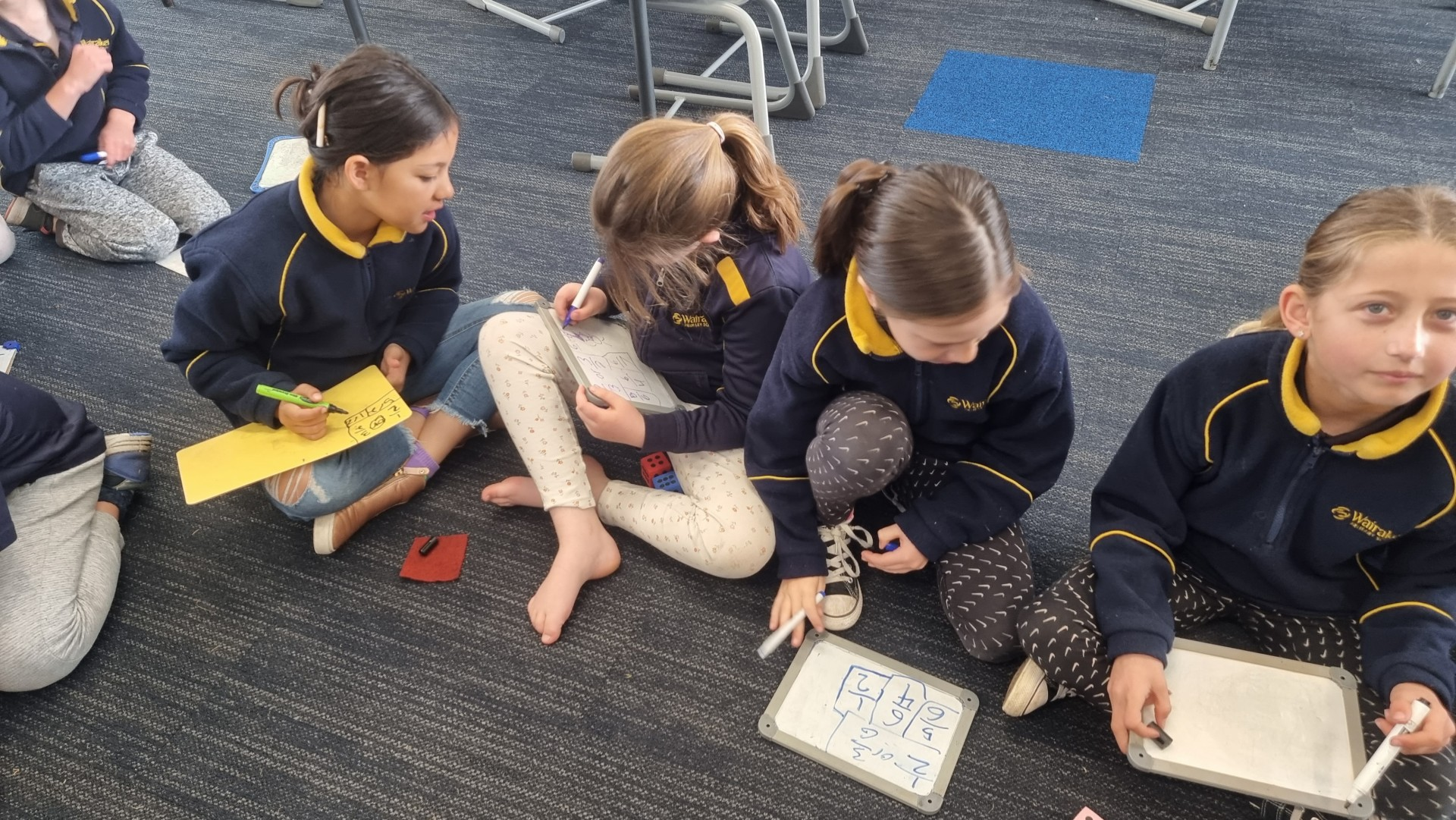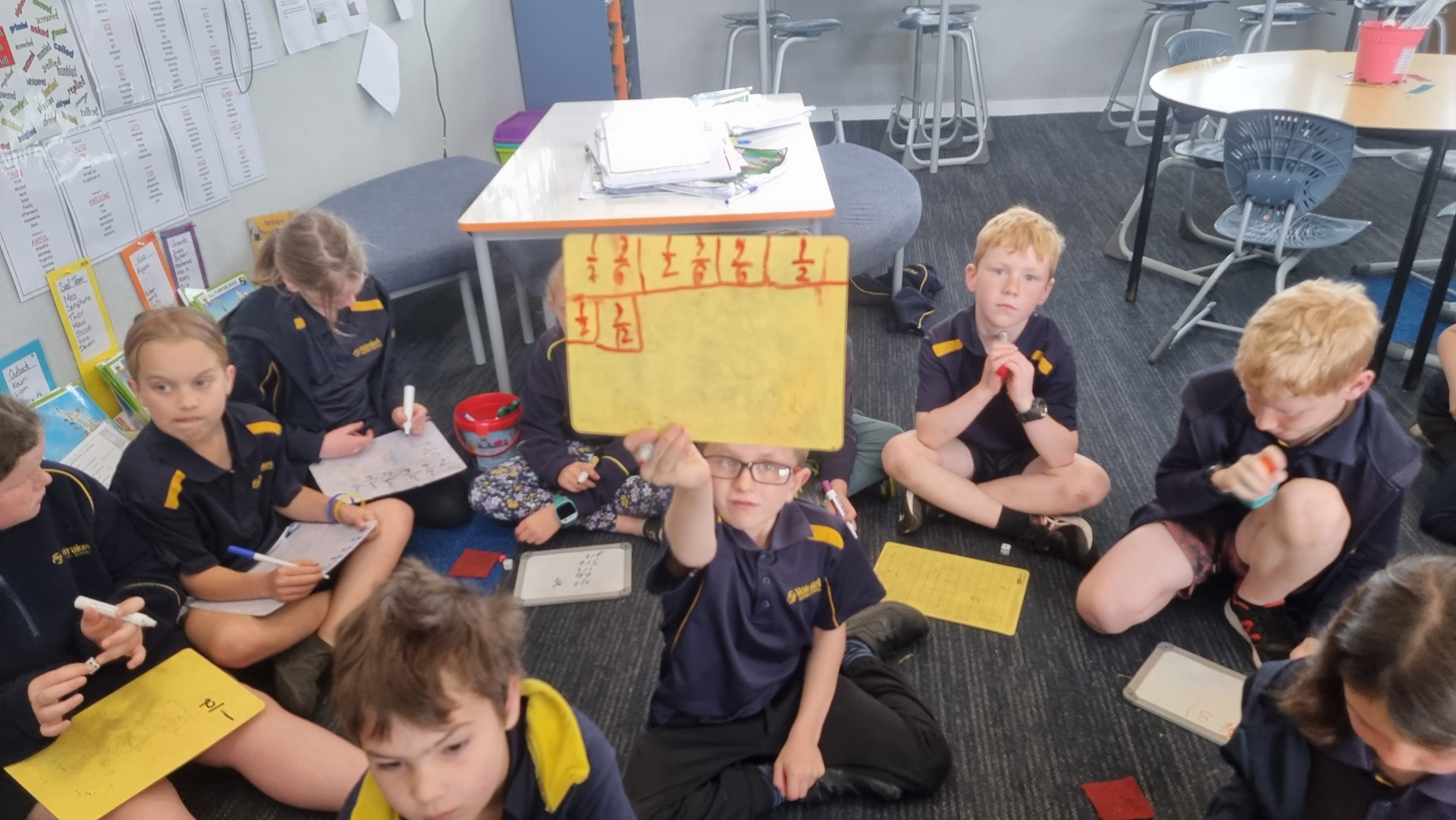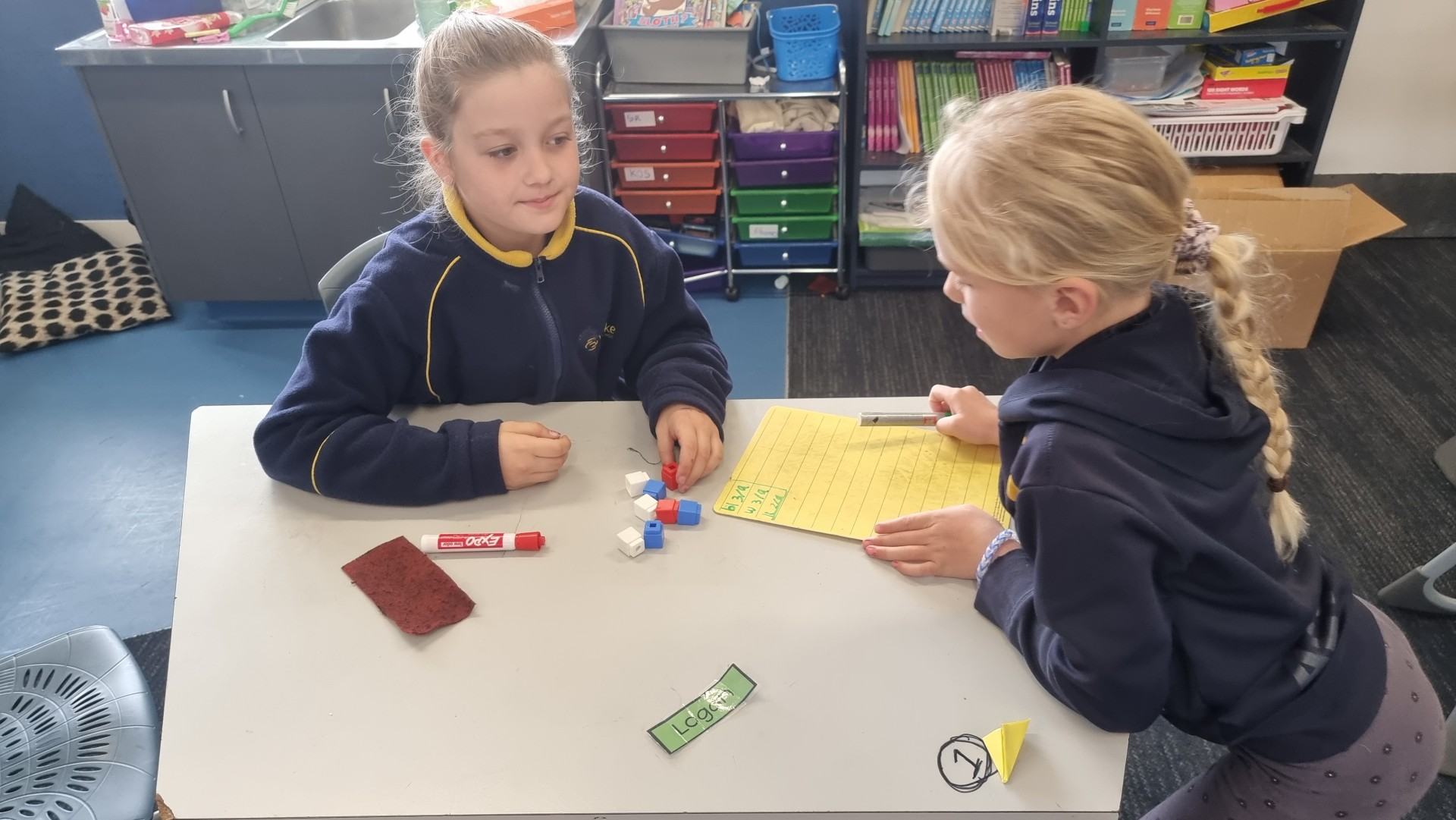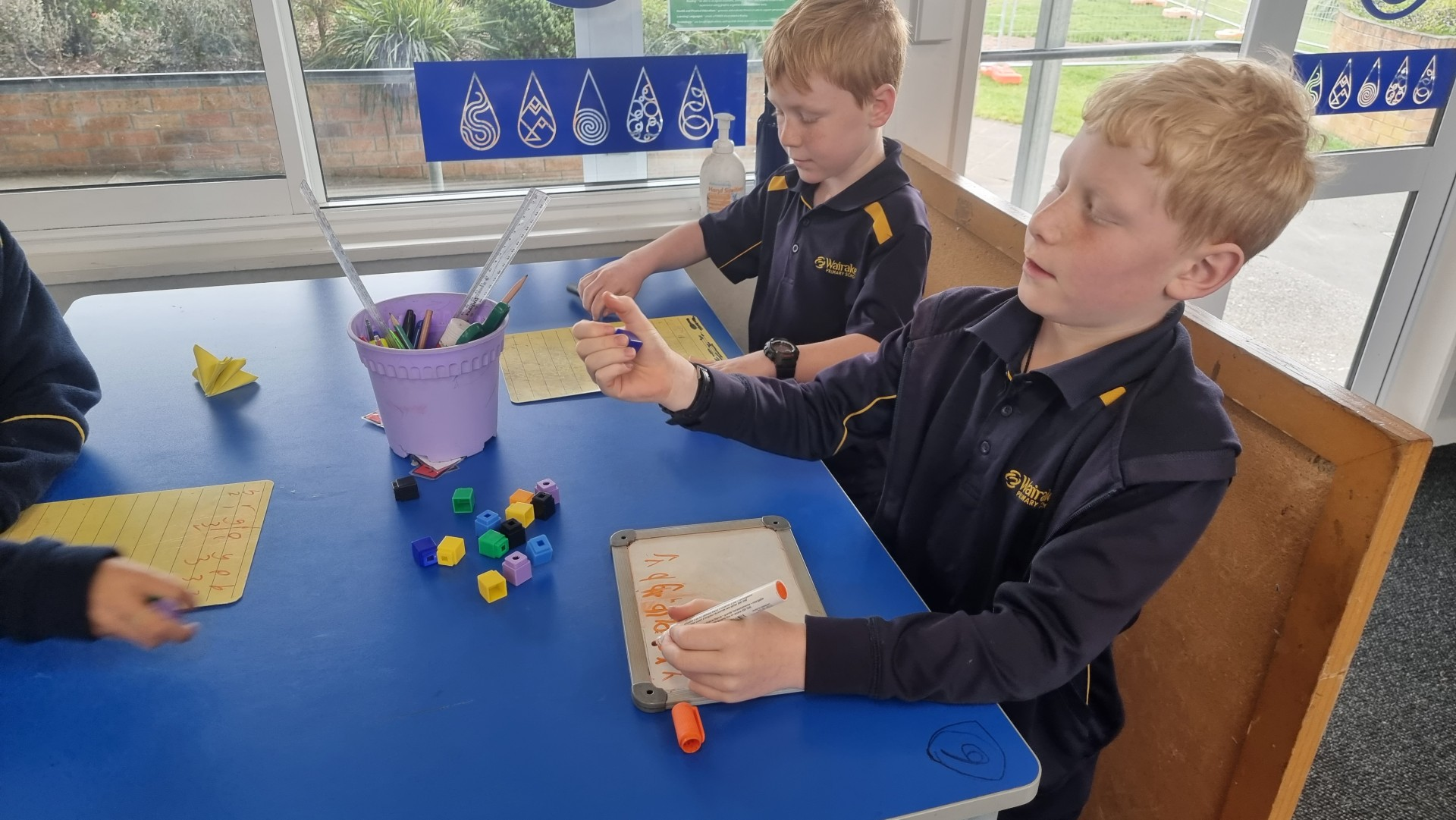# Probability with Fractions

I roll a die - what is the probability that I will roll a five? What about an odd number? What about a number less than four?

If I roll two dice -  How does this change the fractions? What is the probability of rolling an odd number now? What is the probability of rolling a total of seven?

These are just some of the questions that were explored during our probability learning. Being able to find fractions, simplify those fractions and recognise what the keywords are in the questions has been an interesting learning experience for the students of Room 1.Discussions with classmates and exploring collections of blocks was another way that probability was explored. Moving about the classroom with a whiteboard to record results on, the students were able to talk with their peers about their discoveries and help each other with simplifying fractions and checking for answers.